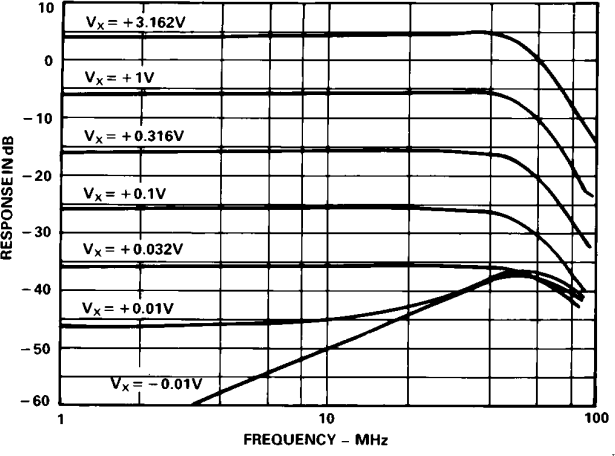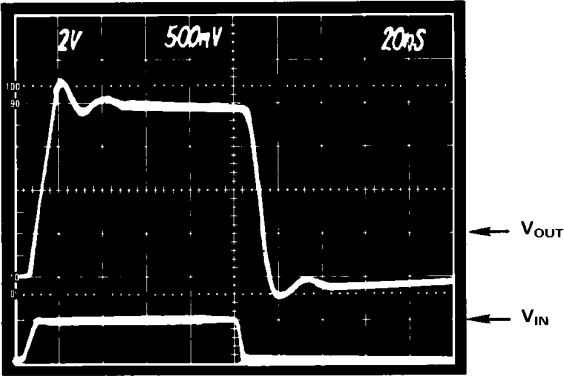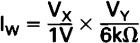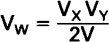# AN-213: Low-Cost, Two-Chip Voltage-Controlled Amplifier and Video Switch

### Introduction

Historically, it has been very difficult to build wide bandwidth, high quality, voltage-controlled amplifiers. Discrete designs have required a great deal of design effort while monolithic or hybrid integrated circuit approaches to VCA’s have been expensive, or they suffered from poor performance.

With the introduction of the AD539JN, a 60MHz analog multiplier in a plastic package, wideband gain-control is now practical at low cost. Used in conjunction with the 5539N wideband op-amp available from either Analog Devices or Signetics Corp. (also in a plastic package), the two devices can be connected together to create a high-speed voltage-controlled amplifier (VCA) or Video Switch with outstanding performance. In addition to providing a 50MHz bandwidth, this combination also satisfies even the most stringent differential phase and differential gain requirements. The AD539/5539 is able to drive a 75Ω terminated coaxial cable directly.

### A Review of Some Basics

Before describing the complete circuit, some basic principles of analog multiplication, as well as the main features of the AD539, will be reviewed. Monolithic multipliers have been commercially available since 1970, following the discovery of an important circuit design technique based on the logarithmic relationship between the base-emitter voltage of a bipolar transistor and its collector current. All IC multipliers now use this concept, known as the translinear principle.

One-quadrant multipliers accept input voltages at VX and VY of only one polarity; accordingly, their operation is confined to quadrant 1 of a 4 quadrant X, Y coordinate system. This type of multiplier is of most use in high-accuracy computational roles.

Two-quadrant multipliers can accept voltages of either polarity at one of their input ports, but they can accept only a single polarity voltage at the other input. In gain-control applications, the bipolar input is considered the “signal” input and the single polarity input is referred to as the “control” input; therefore when VX is restricted to positive values, multiplier operation is confined to Quadrants 1 and 4.

Four-quadrant multipliers allow operation in all four quadrants with any combination of input polarity. Since this variety always preserves the correct sign at the output. It may at first seem that the four-quadrant type would always be the most useful variety of multiplier. However, this is not the case.

Until recently, the main emphasis in improving multiplier performance was directed toward higher precision, and four-quadrant operation was standard. However, the introduction of the AD539 two-quadrant multiplier deviates from this trend, by providing a 50MHz low distortion device optimized for gain control applications.

Four-quadrant analog multipliers have often been used in fast computational applications, in correcting the distortion of wide-angle CRT deflection systems, and in performing modulation and demodulation operations. However, in gain-control applications a two-quadrant multiplier is the better choice because this device is optimized for AC signals. This type of multiplier is often used for precision AGC, for implementing voltage-controlled amplifiers, and for creating various types of programmable filters.

Two-quadrant multipliers such as the AD539 have important advantages in gain-control applications, where there is no need (and it is undesirable) to respond to a bipolar control input voltage. Therefore, one functional advantage of the two-quadrant multiplier is that the control channel can be fully blocked for all values of VX below zero. As a practical matter, the offset voltage of the control channel can be made to be about one-tenth that of a general-purpose four-quadrant multiplier; this also provides improved low level gain accuracy.

Other advantages relate to improvements made possible in the design of the IC when the four-quadrant requirement is removed. In the AD539, these result in higher bandwidth (60MHz versus 1MHz for a general-purpose device) with much smaller signal feedthrough at low gains, better phrase response, lower signal-path distortion (the AD539 will generate less than 0.05% THD at full output in most applications), higher control-channel linearity, and lower noise (particularly at low gains).

### Two-Signal Channels with Common Control

A unique feature of the AD539 is its own separate signal input channels, VY1 and VY2, each simultaneously controlled by a common input, VX. VX has a range of zero to + 3V FS. All inputs are referred to a common (input) ground connection.

The two-signal channels may be used in many different ways. First, of course, they can be used to control the magnitude of a pair separate input signals. The excellent gain-tracking and high separation between channels of the AD539 proves to be valuable in this application; in fact, the bandwidth, crosstalk and other limitations occurring at high frequencies are caused more by the PC board layout than by the IC itself.

In applications where only a single channel is involved, the signal inputs and outputs may be connected in parallel. When driving grounded resistive loads, this configuration has the advantage of increasing the load power by a factor of four. Alternatively, the two-signal channels may be driven from complimentary (phase and antiphase) signals, to achieve distortion figures as low as 0.01%; this mode is generally more utility in low-speed applications (those with less than 1MHz bandwidth).

The two-signal channels may also be connected in series, thus providing a VX2 VY function. This results in a circuit which has higher gain with twice the gain-control range (up to 100dB is practical) or instead, to provide a circuit with a more constant bandwidth over a reduced control voltage range. With the constant bandwidth circuit, the gain now varies as the square if the control voltage, which in some applications is advantageous.

### A 50MHz Voltage-Controlled Amplifier

Figure 1 is a circuit for a 50MHz voltage-controlled amplifier (VCA) suitable for use in high-quality-video-speed-applications. The outputs from the two-signal channels of the AD539 (see “Inside the AD539” for a more complete circuit analysis) are applier to the op-amp in a subtracting configuration. This connection has two main advantages: first, it results in better rejection of the control voltage, particularly when over-driven (VX<0 or VX>3.3V). Secondly, it provides a choice of either non-inverting or inverting responses, using either inputs VY1 or VY2 respectively. In this circuit, the output of the op-amp will equal:Hence, the gain is unity at Vx = + 2V. Since VX can over-range to + 3.3V, the maximum gain in this configuration is about 4.3dB. (Note: If pin 9 of the AD539 is grounded, rather than connected to the output of the 5539N, the maximum gain becomes 10dB.)

The bandwidth of this circuit is over 50MHz at full gain, and is not substantially affected at lower gains. Of course, when VX is zero (or slightly negative, to override the residual input offset) there is still a small amount of capacitive feedthrough at high frequencies; therefore, extreme care is needed in laying out the PC board to minimize this effect. Also, for small values of VX, the combination of this feedthrough with the multiplier output can cause a dip in the response where they are out of phase. Figure 2 shows the AC response from the non-inverting input, with the response from the inverting input, VY2, essentially identical. Test conditions: VY1 = 0.5V RMS for values of VX from + 10mV to + 3.16V; this is with a 75Ω load on the output. The feedthrough at VX = −10mV is also shown.Figure 2. AC Response of the VCA at Different Gains VY = 0.5V RMS.

The transient response of the signal channel at VX = + 2V, VY = VOUT = + or − 1V is shown in Figure 3; with the VCA driving a 75Ω load. The rise and fall-times are both approximately 7ns.Figure 3. Transient Response of the Voltage-Controlled Amplifier VX = + 2 Volts = ± 1 Volt.

In video applications, it is important that the gain and phase of the signal channels remain constant over the full-signal window. These aspects of the response are known as the differential-gain and differential-phase characteristics respectively, and are measured by superimposing a small AC signal at the subcarrier frequency (about 3.58MHz for NTSC systems) on top of a bias signal that modulates the channel over its nominal range, usually 0 to + 1V. Figure 4 shows the variation in gain for VY = − 1V to + 1V at a frequency of 3.58MHz, for three values of VX. Figure 5 shows the phase variation under the same conditions. In most respects, this performance is similar to that which may be achieved using more expensive custom circuitry. Although the control channel of the AD539 can be more easily overloaded by a rapidly-changing-step input.

A few final circuit details: in general, the control amplifier compensation capacitor for pin 2, CC, must have a minimum value of 3000pF (3nF) to provide both circuit stability and maximum control bandwidth. However, if the maximum control bandwidth is not needed, then it is advisable to use a larger value of CC, with typical values between 0.01 and 0.1µF. Like many aspects of design, the value of CC will be a tradeoff: higher values of CC will lower the high frequency distortion, reduce the high frequency crosstalk, and improve the signal channel phase response. Conversely, lower values of CC will provide a higher control channel bandwidth at the expense of degraded linearity in the output response when amplitude modulating a carrier signal. The Control channel bandwidth will vary in inverse proportion to the value of CC, providing a typical bandwidth of 2MHz with a CC of 0.01µF and a VX voltage of + 1.7 volts.

Both the bandwidth and pulse response of the control channel can be further increased by using a feedforward capacitor, CFF, with a value between 5 and 20 percent of CC. CFF should be carefully adjusted to give the best pulse response for a particular step input applied to the control channel. Note that since CFF is connected between a linear control input (pin 1) and a logarithmic node, the settling time of the control channel with a pulse input will vary with different control input step levels.

Diode D1 clamps the logarithmic control node at pin 2 of the AD539, (preventing this point from going too negative); this diode helps decrease the circuit recovery time when the control input goes below ground potential.

### The AD539/5539 Combination as a Fast, Low Feedthrough, Video Switch

Figure 6 shows how the AD539/5539 combination can be used to create a fast video speed switch suitable for many high frequency applications including color key switching. It features both inverting and non-inverting inputs and can provide an output of ± 1V into a reverse-terminated 75Ω load (or ± 2V into 150Ω). An optional output offset adjustment is provided. The input range of the video switch is the same as the output range: ± 1V at either input generates ± 1V (noninverting) or ± 1V (inverting) across the 75Ω load. The circuit provides a dimensionless gain of about 1, when “ON”, or zero when “OFF”.

The differential configuration uses both channels of the AD539 not only to provide alternative input phases, but also to eliminate the switching pedestal due to step-changes in the output current as the AD539 is gated on or off.

The waveforms shown in Figures 7 and 8 were taken across a 75Ω termination; in both photos, the signal of 0 to + 1V (in this case, an offset sine wave at 1MHz) was applied to the non-inverting input. In Figure 7, the envelope response shows the output being fully switched in about 50ns. Note that the output in ON when the control input is zero (or more negative) and OFF for a control input of + 1V or more. There is very little control-signal breakthrough.

Figure 8 shows the response to a pulse of 0 to + 1V on the signal channel. With the control input held at zero, the rise-time is under 10ns. The response from the inverting input is similar.

The differential-gain and differential-phase characteristics of this switch are compatible with video applications. The incremental gain changes less than 0.05dB over a signal window of 0 to + 1V, with a phase variation of less than 0.5 degree at the subcarrier frequency of 3.58MHz. The noise level of this circuit measured at the 75Ω load is typically 200µV in a 0 to 50MHz bandwidth or approximately 100nV per root hertz. The noise spectral density is essentially flat to 40MHz.

Figure 9 is a simplified schematic outlining the main design features of the AD539 multiplier. Q1 through Q6, which from the translinear core of the multiplier, are multi-emitter NPN transistors having a very low base resistance, to minimize noise and distortion; emitter area scaling is also used in optimizing this crucial section of circuit. Each of the pairs Q1-Q2, Q3-Q4, and Q5-Q6 from what is called a “controlled cascade” circuit; this is basically a grounded-base transistor to which has been added another device which removes some of the signal from the emitter. This alters the gain of the cascade, from almost unity (when no current is removed) to zero (when all the signal is removed.) The “controlled cascade” configuration has very desirable characteristics for use in two-quadrant multiplication.

A stable 1.375mA reference current (which determines the multiplier scaling) is supplied to the common emitters of the controlled-cascode Q1-Q2, whose bases are biased by the control amplifier (a high-speed op-amp). When the control input VX is zero, Q2 is biased off. This bias voltage is conveyed to Q3 and Q6, which are likewise turned off; signal transmission to the outputs is thus blocked. As VX increases, the current through RX (IX) is forced to flow in the collector of Q2; this current only represents a fractional part of the 1.375mA reference current. When VX = 3V (its nominal full-scale value), 1.2mA flows in RX and Q2; this is 0.873 (or 87.3%) of the reference current.

Correspondingly, the same fraction of the signal and bias-currents that is supplied to the common emitter nodes controlled cascodes Q3-Q4 and Q5-Q6 is conveyed to the two outputs.

Now consider the signals paths. The voltages VY1 and VY2 are converted to currents by V-I converters which have a transresistance of 1.74Ω. At full-scale input of ± 2V, the signal current supplied to the cascodes is ± 1.15mA; this is superimposed on a bias current of 2.75mA. Thus, when VX = + 3V, the collector currents of either Q3 or Q6 will consist of a signal component of ± 1mA (0.873 × 1.15mA) and a DC component of 2.4mA, both of these currents being proportionally less for other values of VX. The DC component is removed by resistors RX1 and RX2, driven directly from VX. The final output is thus a current of value:Note that the peak value of VY can be ± 4.2V (using a − VS supply of at least − 7.5V) and VX can overrange by 10% to + 3.3V, so the peak output current of each channel can be slightly more than ± 2mA, for maximum of ± 4mA when the channels are used in parallel. These currents may be delivered directly to grounded load resistors or to terminated coaxial cables. With coaxial cables, the full 60MHz bandwidth of the AD539 can be realized, but the peak signal amplitude will be quite limited (to only ± 330mV using a 75Ω load). Clearly, some additional gain is needed.

Unfortunately, the amplifiers necessary for additional gain could not be included on the AD539, due mainly to power dissipation considerations. Also, gain errors (of up to ± 1.5dB) will occur using a simple load resistor, because of the 20 percent tolerance of the thin-film resistors. Fortunately, by using external op-amps, the output currents may be converted to much larger voltages, using on-chip applications resistors RW and RZ provided for this purpose. These resistors have a nominal value of 6kΩ. But they are laser-trimmed during manufacture so as to result in high gain-accuracy when used as the feedback resistors around an inverting op-amp. When using just RW (RW1 for CH1, RW2 for CH2), the transfer characteristic becomes:When RW and RZ are used in parallel, the gain is halved, that is:The bandwidth is now largely determined by the op-amp. For wideband applications, the 5539N is an ideal low-cost complement to the AD539; this combination is capable of providing ± 1V into a 75Ω load with only a very small degradation of the 60MHz bandwidth achieved by the AD539 alone.

### Layout of Video-Bandwidth Circuitry

Careful component layout, adequate power supply bypassing, and proper coaxial cable termination are all very important in the implementation of video-bandwidth circuits in general. Unfortunately, even when these precautions are taken, some added difficulties can still arise in the case of voltage-controlled amplifiers. This can happen when leakage of the input signal to the output occurs when the gain should be zero. This feedthrough will cause ghost images which are generated by the high-frequency components of the unwanted signal.

A good ground plane is essential! To help assure this, it is recommended that part of this ground be run between the rows of the pins of both chips, on both the upper and lower surfaces of the PC board. The ground plane for a typical AD539/5539 layout is shown in Figure 10. In addition, all decoupling capacitors must have minimum lead lengths to this ground plane. Also, the input and output connections must be kept short, and should be physically separated as far as possible from each other. Separate power supply decoupling for the AD539JN and 5539N is also recommended.